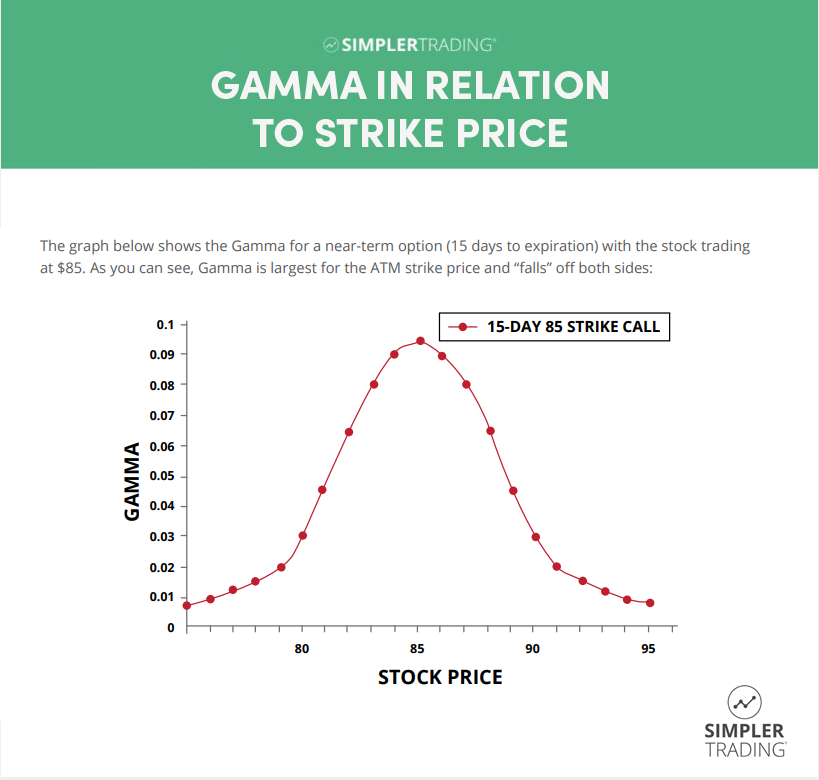# What Is Gamma In Options?

## What is gamma in options?

Gamma is one of those concepts in options trading that can seem intimidating at first, but it’s not difficult to understand. Did you know that gamma affects every option trade you make? It’s important to understand why that is. So what exactly is gamma, and why should you care about the greeks? Keep reading to find out!

### Key Takeaways

• Options gamma is a measure of the rate of change in the options delta in relation to the underlying asset price.
• Positive Gamma means that the delta will increase as the underlying asset price increases and a negative gamma means that the delta will decrease as the underlying asset price increases.
• Gamma tells us how much the delta will change for each one-point move in the underlying asset price

## What is the option’s gamma and why is it important?

Options gamma measures the rate of change in the options delta in relation to the underlying asset price. Simply put, it tells us how much the delta will change for each one-point move in the underlying asset price. Gamma is important because it helps us to measure and manage risk. For example, when implied volatility is high, options prices tend to be more volatile, and therefore options gamma is also high. This means that the deltas of our options positions will also be more volatile, and we need to be aware of this when managing our risk.

Gamma can be either positive or negative; this tells us whether an options contract delta will increase or decrease as the underlying asset price changes. Positive gamma means that the delta will increase as the underlying asset price increases (i.e., the option position becomes more “long”). Negative gamma on a short call means that the delta will decrease as the underlying asset price increases (i.e., the option position becomes more “short”).

If a trader is long on options, having positive gamma will positively affect the options contract’s value as the underlying asset price increases.

This is because, all else being equal, an increasing underlying asset price should mean that our option position will make money. Of course, there are exceptions to this rule (for example, if we are short a call option with a negative gamma), but in general, traders want positions to have positive gamma. There are several ways to trade options with a positive gamma, which will be covered in more detail in future articles.

## How is gamma used in options trading

### Speculating

When trading stock options, gamma should be noted. When a trader buys either calls or puts, they will add positive gamma value to their overall position. Writing calls and puts, or selling-to-open, traders will add negative gamma to their overall position. Why is this important? Simply put, this will tell how the value of your change trades as it moves further in-to-the-money or (ITM) or conversely as you move out-of-the-money.### Hedging

Gamma is also commonly used by traders who are hedging a position. When using gamma to hedge a long position, having lower gamma will result in your position being less affected by movements in the underlying security.

## Gamma and theta

It’s worth mentioning the relationship between gamma and theta. Typically if an options contract has high theta, it will also have high gamma. This means that an options contract potentially higher profits if the underlying security moves quickly in the right direction. Unfortunately, high theta means that the extrinsic value of the contract will decay faster.

If the movement in the underlying asset doesn’t happen quickly, you may be subject to substantial losses as the contract approaches expiration.

## Why is gamma the same for calls and puts?

Gamma is positive for both long calls and puts. This is because, as the underlying asset’s price increases, the value of the call contract increase at a greater rate than the underlying asset’s price (and vice versa for puts). Gamma is the same for calls and puts because implied volatility is a measure of the underlying asset’s volatility and not of the options themselves. Therefore, since implied volatility is constant, so is gamma.

## Conclusion

So as you can see, the greeks are an integral part of options trading. If you truly want to trade options profitably, having a nuanced understanding of the greeks will help you make informed decisions when choosing strike prices and structuring trades.